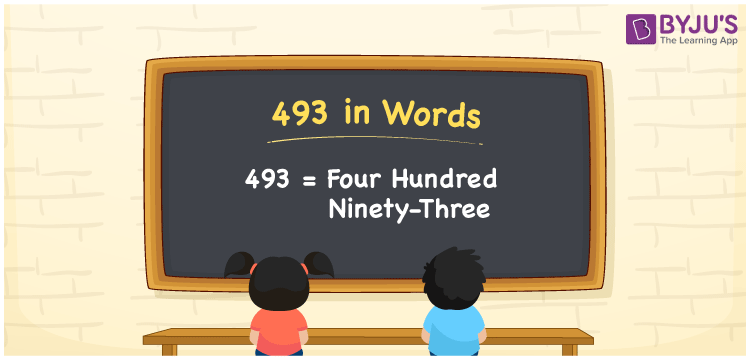# 493 in Words

We can write 493 in words as Four hundred ninety-three. It is easy to derive the word form of the number 493 using a place value chart. Also, we use the word form of the number to express or spell that particular number. Suppose you paid a bill of Rs. 493 in a stall, then you can say, “I paid Four hundred ninety-three rupees bill in the stall”. Also, note that 493 is a cardinal number since it denotes a specific amount.

 493 in words Four hundred ninety-three Four hundred ninety-three in Numbers 493

## 493 in English Words

We generally write numbers in words using the English alphabet. Thus, we spell 493 in English words as “Four hundred ninety-three”.## How to Write 493 in Words?

As we can see, 493 is a three-digit number we should make a place value chart with three columns, as given below.

 Hundreds Tens Ones 4 9 3

Here, ones = 3, tens = 9, hundreds = 4

Let us write these digits as per their place values.

4 × Hundred + 9 × Ten + 3 × One

= 4 × 100 + 9 × 10 + 3 × 1

= 400 + 90 + 3

= Four hundred + Ninety + Three

= Four hundred ninety-three

Therefore, 493 in words = Four hundred ninety-three

493 is a natural number that precedes 494 and succeeds 492.

493 in words – Four hundred ninety-three

Is 493 an even number? – No

Is 493 an odd number? – Yes

Is 493 a perfect square number? – No

Is 493 a perfect cube number? – No

Is 493 a prime number? – No

Is 493 a composite number? – Yes

## Frequently Asked Questions on 493 in Words

Q1

### How do you write 493 in word form?

The word form of the number 493 is “Four hundred ninety-three”.
Q2

### How to write Rs. 493 in words on a cheque?

On a cheque, we generally write Rs. 493 in words as “Four hundred ninety-three rupees only”.
Q3

### Write the expanded notation of 493 in words.

The expanded notation of 493 in words = 400 + 90 + 3 = Four hundred + Ninety + Three